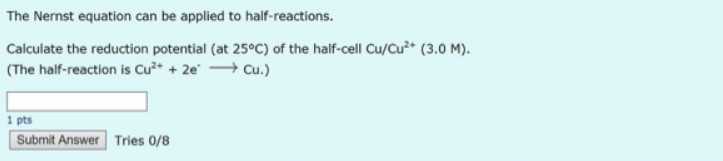# Problem: The Nernst equation can be applied to half-reactions. Calculate the reduction potential (at 25°C) of the half-cell Cu/Cu2+. (3.0 M). (The half-reaction is Cu2+ + 2e- → Cu.)

###### FREE Expert Solution
80% (410 ratings)###### Problem Details

The Nernst equation can be applied to half-reactions.

Calculate the reduction potential (at 25°C) of the half-cell Cu/Cu2+. (3.0 M).

(The half-reaction is Cu2+ + 2e- → Cu.)